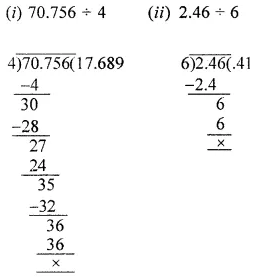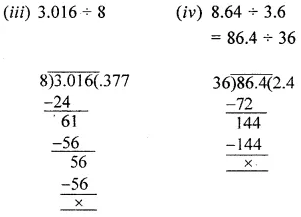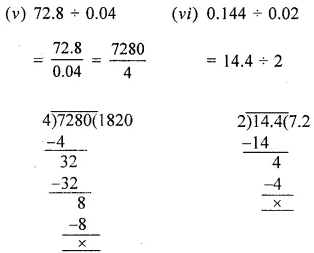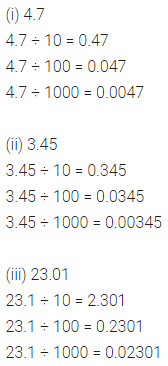# ML Aggarwal Class 6 Solutions for ICSE Maths Chapter 7 Decimals Ex 7.4

## ML Aggarwal Class 6 Solutions for ICSE Maths Chapter 7 Decimals Ex 7.4

Question 1.
Evaluate the following:
(i) 3.7 × 4.5
(ii) 12.08 × 9.3
(iii) 238.06 × 7.5
(iv) 0.79 × 32.4
(v) 3.6 × 1.4 × 0.7
(vi) 9.01 × 2.5 × 1.6
Solution:Question 2.
Work out the following:
(i) 70.756 ÷ 4
(ii) 2.46 ÷ 6
(iii) 3.016 ÷ 8
(iv) 8.64 ÷ 3.6
(v) 72.8 ÷ 0.04
(vi) 0.144 ÷ 0.02
Solution:Question 3.
Multiply each of the following numbers by 10, 100 and 1000 orally ;
(i) 4.7
(ii) 3.45
(iii) 23.01
Solution:Question 4.
Divide each of the following numbers by 10, 100 and 1000 (orally):
(i) 4.7
(ii) 3.45
(iii) 23.01
Solution: